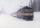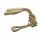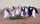Two squares

Two squares whose sides are in the ratio 5:2 have sum of its perimeters 73 cm. Calculate the sum of area this two squares.

Result

S1+S2 =  197.1 cm2

Solution:Leave us a comment of example and its solution (i.e. if it is still somewhat unclear...):Be the first to comment!To solve this verbal math problem are needed these knowledge from mathematics:

Do you have a linear equation or system of equations and looking for its solution? Or do you have quadratic equation?

Next similar examples:

1. Garden plotCalculate how many meters of fence need to fence the square garden with length and width of 22 meters.
2. TrainThe train passes part of the line for 95 minutes at speed 75 km/h. What speed would have to go in order to shorten the driving time of 20 minutes?
3. Perimeter of squareThe square has a circumference 17cm. What is its area?
4. RunnersFor three runners (on the first to third place) is prepared 30 chocolate that they be distributed in the ratio of 3 : 2 : 1 How much chocolate will get everyone?
5. QuizTested student answered correctly on a 4/ 8 questions. Wrong answers was 16. How many questions answered?
6. NormThree workers planted 3555 seedlings of tomatoes in one dey. First worked at the standard norm, the second planted 120 seedlings more and the third 135 seedlings more than the first worker. How many seedlings were standard norm?
7. Equation 29Solve next equation: 2 ( 2x + 3 ) = 8 ( 1 - x) -5 ( x -2 )
8. Unknown number 11That number increased by three equals three times itself?
9. Dropped sheetsThree consecutive sheets dropped from the book. The sum of the numbers on the pages of the dropped sheets is 273. What number has the last page of the dropped sheets?
10. Eq1Solve equation: 4(a-3)=3(2a-5)
11. BenchesThe park has 64 benches. Occupied are by 18 more than empty. How many benches are occupied and empty ?
12. Find xSolve: if 2(x-1)=14, then x= (solve an equation with one unknown)
13. Forest nurseryIn the forest nursery after winter, they found that 1/10 stems died out of them. For them, they land 193 new spruces. How many spruces are in the forest nursery?
14. Simple equationSolve the following simple equation: 2. (4x + 3) = 2-5. (1-x)
15. ToysThe toy shop sold the same number of balls for 62 CZK and skipping ropes for 34 CZK. They income was 576 CZK. How many sold balls and how many skipping ropes?
16. Simple equationSolve for x: 3(x + 2) = x - 18
17. Rabbit familyRabbit family ate 32 pieces of carrots, small one ate 6 pieces, dad 5 more than the mother. How much ate mom?